Test: Signals & Systems - 2

# Test: Signals & Systems - 2

Test Description

## 20 Questions MCQ Test GATE ECE (Electronics) 2023 Mock Test Series | Test: Signals & Systems - 2

Test: Signals & Systems - 2 for Electronics and Communication Engineering (ECE) 2023 is part of GATE ECE (Electronics) 2023 Mock Test Series preparation. The Test: Signals & Systems - 2 questions and answers have been prepared according to the Electronics and Communication Engineering (ECE) exam syllabus.The Test: Signals & Systems - 2 MCQs are made for Electronics and Communication Engineering (ECE) 2023 Exam. Find important definitions, questions, notes, meanings, examples, exercises, MCQs and online tests for Test: Signals & Systems - 2 below.
Solutions of Test: Signals & Systems - 2 questions in English are available as part of our GATE ECE (Electronics) 2023 Mock Test Series for Electronics and Communication Engineering (ECE) & Test: Signals & Systems - 2 solutions in Hindi for GATE ECE (Electronics) 2023 Mock Test Series course. Download more important topics, notes, lectures and mock test series for Electronics and Communication Engineering (ECE) Exam by signing up for free. Attempt Test: Signals & Systems - 2 | 20 questions in 60 minutes | Mock test for Electronics and Communication Engineering (ECE) preparation | Free important questions MCQ to study GATE ECE (Electronics) 2023 Mock Test Series for Electronics and Communication Engineering (ECE) Exam | Download free PDF with solutions
 1 Crore+ students have signed up on EduRev. Have you?
Test: Signals & Systems - 2 - Question 1

### If relation between input x[n] and output y[n] of a system is given by y[n] - 1/2 y[n -1] = x[n] then the system is

Detailed Solution for Test: Signals & Systems - 2 - Question 1

Simply lake Z transform from both sides:

Then H(z)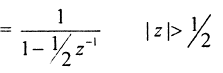it is right of the right - most pole

Test: Signals & Systems - 2 - Question 2

### lf x[n] = - u[-n -1] + (1 /2)n u[-n -1] then ROC of signal is:

Detailed Solution for Test: Signals & Systems - 2 - Question 2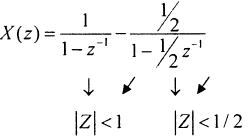Overall ROC is IZI < 1 /2

Test: Signals & Systems - 2 - Question 3

### Consider, discrete time signal x[n] = (1 /3)n u[n] If signal y[n] is defined as y[n] = x[—n], —∞< n < ∞ then value of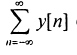equals to

Detailed Solution for Test: Signals & Systems - 2 - Question 3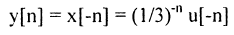Now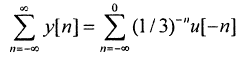Put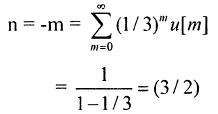Test: Signals & Systems - 2 - Question 4

If signal x(t) and h(t) shown in figure are convolved to yield y(t).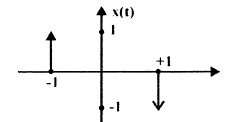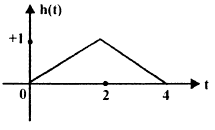Detailed Solution for Test: Signals & Systems - 2 - Question 4

Simply use difference and sum between points.

Test: Signals & Systems - 2 - Question 5

Given that g (t)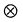g (t) = t exp (—t) u(t) then value of g(t) is

Detailed Solution for Test: Signals & Systems - 2 - Question 5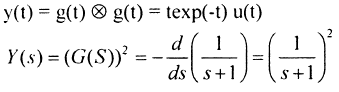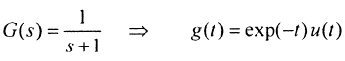Test: Signals & Systems - 2 - Question 6

If u(t) r(t) denotes unit step and unit ramp respectively then u(t)r(t) denotes their convolution, then function u(t + 1)r(t — 2) is given by.

Detailed Solution for Test: Signals & Systems - 2 - Question 6

y(t) = u(t)r(t)

Y(s) = 1/s3

Test: Signals & Systems - 2 - Question 7

If wave form is given only for I period then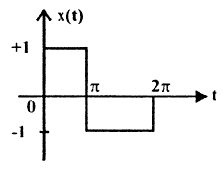Q. Waveform x (t) is

Test: Signals & Systems - 2 - Question 8

If wave form is given only for I period thenQ. Fourier series representation on of x (t) will be.

Detailed Solution for Test: Signals & Systems - 2 - Question 8

it is an odd function with half symmetry.

Test: Signals & Systems - 2 - Question 9

If complex Fourier- series coefficient Ck is given as Ck = (— 1/2)|k| then value of x(t) is:

Test: Signals & Systems - 2 - Question 10

Which statements are true about Fourier transform?

1. If x(t) is real then X(jω) is complex conjugate symmetric.
2. If x(t) is imaginary then X(jω) is complex conjugate anti-symmetric
3. If x(t) is even then X(jω) is also even.
Test: Signals & Systems - 2 - Question 11

Check status of linearity of given functions.

I .   x1(t) = x(t) .cost

2.  x2(t) = cos(x(t)).t

Detailed Solution for Test: Signals & Systems - 2 - Question 11

x2(t) = t cos (x (t)

if x(t) = 0   ⇒  x2(t) = t

So x2(t) is non - linear

Test: Signals & Systems - 2 - Question 12

If Fourier transform of x(t) is X(ω) then Fourier transform of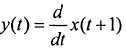Detailed Solution for Test: Signals & Systems - 2 - Question 12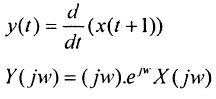Test: Signals & Systems - 2 - Question 13

0.5 If Z-Transform X(z) of a sequence x[n] is given by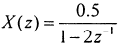.It is given that R.O.C. of X (z) includes unit circle then

Q. Value of x is

Detailed Solution for Test: Signals & Systems - 2 - Question 13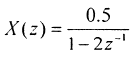Test: Signals & Systems - 2 - Question 14

0.5 If Z-Transform X(z) of a sequence x[n] is given by.It is given that R.O.C. of X (z)includes unit circle then

Q. In above system if x[n] is causal then value of x is

Detailed Solution for Test: Signals & Systems - 2 - Question 14

if it includes, unit - circle then, Iz| < 2

x[n] = - (0.5) 2n u(-n-1)

x = 0

if it is causal then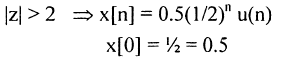Test: Signals & Systems - 2 - Question 15

If frequency response of a causal & stable linear Time Invariant system is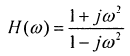, then value of phase delay and group delay are.

Detailed Solution for Test: Signals & Systems - 2 - Question 15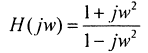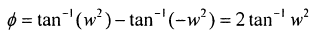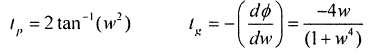Test: Signals & Systems - 2 - Question 16

Which among the following are the stable discrete time systems?

1. y(n) = x(4n)
2. y(n) = x(-n)
3. y(n) = ax (n) + 8
4. y(n) = cos x(n)

*Answer can only contain numeric values
Test: Signals & Systems - 2 - Question 17

For given Fourier — series coefficients for real signals Co = 1, C1 = — 2, C2 = — 1 then power of this signal is _______

Test: Signals & Systems - 2 - Question 18

What is inverse Fourier transform of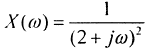Detailed Solution for Test: Signals & Systems - 2 - Question 18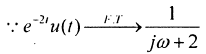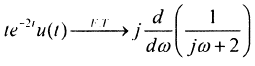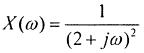Test: Signals & Systems - 2 - Question 19

Which statement is true about y[n] = x[n] . cos (ωo n)

*Answer can only contain numeric values
Test: Signals & Systems - 2 - Question 20

For given signal s(t), shown below what is slope of matched filter output during interval 1 ≤ t ≤ 2 is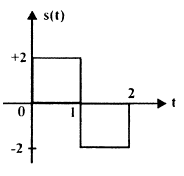Detailed Solution for Test: Signals & Systems - 2 - Question 20

h(t) =    = s(2

y(t) = NWh(t )

Just draw y(t) and then calculate slope of y(t)

## GATE ECE (Electronics) 2023 Mock Test Series

21 docs|263 tests
Information about Test: Signals & Systems - 2 Page
In this test you can find the Exam questions for Test: Signals & Systems - 2 solved & explained in the simplest way possible. Besides giving Questions and answers for Test: Signals & Systems - 2, EduRev gives you an ample number of Online tests for practice

## GATE ECE (Electronics) 2023 Mock Test Series

21 docs|263 tests(Scan QR code)